ALL

Page: (Previous)   1  2  3  4  (Next)
ALL

### M

#### MAT-HS.F-IF.06

 MAT-HS Targeted Standards(F) Concept: Function(IF) Domain: Interpreting FunctionsCluster: Interpret functions that arise in applications in terms of the context MAT-HS.F-IF.06 Calculate and interpret the average rate of change of a function (presented symbolically or as a table) over a specified interval. Estimate the rate of change from a graph.

## Student Learning Targets:

• I can
• I can

### Reasoning Targets

• I can estimate the average rate of change over a specified interval of a function from its graph.

### Skills (Performance) Targets

• I can calculate and interpret the average rate of change of a function presented symbolically or as a table.

• I can
• I can

## Proficiency Scale

 Score Description Sample Activity 4.0 In addition to Score 3.0, the student demonstrates in-depth inferences and applications regarding more complex material that go beyond end of instruction expectations. - 3.5 In addition to Score 3.0 performance, the student demonstrates in-depth inferences and applications regarding the more complex content with partial success. 3.0 “The Standard.” The student demonstrates no major errors or omissions regarding any of the information and processes that were end of instruction expectations. - 2.5 The student demonstrates no major errors or omissions regarding the simpler details and processes (Score 2.0 content) and partial knowledge of the more complex ideas and processes (Score 3.0 content). 2.0 The student demonstrates no major errors or omissions regarding the simpler details and processes but exhibits major errors or omissions regarding the more complex ideas and processes (Score 3.0 content). - 1.5 The student demonstrates partial knowledge of the simpler details and processes (Score 2.0 content) but exhibits major errors or omissions regarding the more complex ideas and procedures (Score 3.0 content). 1.0 With help, the student demonstrates a partial understanding of some of the simpler details and processes (Score 2.0 content) and some of the more complex ideas and processes (Score 3.0 content). - 0.5 With help, the student demonstrates a partial understanding of some of the simpler details and processes (Score 2.0 content) but not the more complex ideas and processes (Score 3.0 content). 0.0 Even with help, the student demonstrates no understanding or skill. -

## Resources

 Web Vocab rate of change interval

#### MAT-HS.F-IF.07

 MAT-HS Targeted Standards(F) Concept: Function(IF) Domain: Interpreting FunctionsCluster: Analyze functions using different representations MAT-HS.F-IF.07 Graph functions expressed symbolically and show key features of the graph, by hand in simple cases and using technology for more complicated cases. a. Graph linear and quadratic functions and show intercepts, maxima, and minima. b. Graph square root, cube root, and piecewise-defined functions, including step functions and absolute value functions. c. Graph polynomial functions, identifying zeros when suitable factorizations are available, and showing end behavior. d. (+) Graph rational functions, identifying zeros and asymptotes when suitable factorizations are available, and showing end behavior. e. Graph exponential and logarithmic functions, showing intercepts and end behavior, and trigonometric functions, showing period, midline, and amplitude. f.  Graph f(x)=sin x and f(x)=cos x as representations of periodic phenomena. g.  (+) Graph trigonometric functions, showing period, midline, phase shift and amplitude

## Student Learning Targets:

• I can
• I can

• I can
• I can

### Skills (Performance) Targets

• I can graph a linear equation.
• I can manipulate the form of a linear equation.
• I can identify a linear equation.
• Given an equation, I can graph a function and label all critical points, e.g. vertex, max or min value, x-intercepts, y-intercepts, slope.
• I can graph functions expressed symbolically and show key features of the graph (graph simple cases by hand and use technology to show more complicated cases).
• I can graph linear functions showing intercepts.
• I can graph quadratic functions showing intercepts, a maximum or a minimum.
• I can graph square root, cube root and piecewise-defined functions, including step functions and absolute value functions.
• I can graph exponential functions, showing intercepts and end behavior. I can graph polynomial functions, identifying zeros when factorable, and show end behavior.

• I can
• I can

## Proficiency Scale

 Score Description Sample Activity 4.0 In addition to Score 3.0, the student demonstrates in-depth inferences and applications regarding more complex material that go beyond end of instruction expectations. -Student is able to determine and describe intervals where the function is increasing and decreasing using interval notation. 3.5 In addition to Score 3.0 performance, the student demonstrates in-depth inferences and applications regarding the more complex content with partial success. 3.0 The student can: interprets and sketches key features of the linear graph (HS.F-IF.7a1) including: intercepts positive/negative slope interprets and sketches key features of the exponential graph (HS.F-IF.7b2) including: end behavior (growth/decay) interprets and sketches key features of the quadratic graph (HS.F-IF.7e) including: minimum/maximum axis of symmetry intercepts construct simple graphs by hand and use technology for more complicated cases. The student exhibits no major errors or omissions. Graph the function and identify the vertex, intercepts, and axis of symmetry.  y=x2-8x-7 Graph the function and identify the end behavior of the graph. y=(1/2)x 2.5 The student demonstrates no major errors or omissions regarding the simpler details and processes (Score 2.0 content) and partial knowledge of the more complex ideas and processes (Score 3.0 content). 2.0 There are no major errors or omissions regarding the simpler details and processes as the student can: recognize and recall basic terminology, such as: linear equation. quadratic equation. exponential equation. slope intercepts minimum maximum end behavior growth and decay symmetry axis of symmetry only interprets and sketches some of the key features of the graph. can identify if a function is linear, exponential, or quadratic However, the student exhibits major errors or omissions regarding the more complex ideas and processes. Graph the set of points and determine the relationship.  (i.e. linear, exponential, or quadratic)   (0, 0), (1, 1), (-1, -0.5), (2, 3) 1.5 The student demonstrates partial knowledge of the simpler details and processes (Score 2.0 content) but exhibits major errors or omissions regarding the more complex ideas and procedures (Score 3.0 content). 1.0 With help, the student demonstrates a partial understanding of some of the simpler details and processes (Score 2.0 content) and some of the more complex ideas and processes (Score 3.0 content). - 0.5 With help, the student demonstrates a partial understanding of some of the simpler details and processes (Score 2.0 content) but not the more complex ideas and processes (Score 3.0 content). 0.0 Even with help, the student demonstrates no understanding or skill. -

## Resources

 Web Vocab intercepts maximum minimum square root function cube root function piecewise-defined function step function absolute value function polynomial function zeroes exponential function

#### MAT-HS.F-IF.07.a

 MAT-HS Targeted Standards(F) Concept: Function(IF) Domain: Interpreting FunctionsCluster: Analyze functions using different representations MAT-HS.F-IF.07* Graph functions expressed symbolically and show key features of the graph, by hand in simple cases and using technology for more complicated cases. Graph linear functions and show intercepts, maxima, and minima where appropriate.

## Student Learning Targets:

• I can
• I can

• I can
• I can

### Skills (Performance) Targets

• I can graph a linear equation and show intercepts. (HS.F-IF.7.a)

• I can
• I can

## Proficiency Scale

 Score Description Sample Activity 4.0 (advanced) In addition to Score 3.0, the student demonstrates in-depth inferences and applications regarding more complex material that go beyond end of instruction expectations. -Student is able to determine and describe intervals where the function is increasing and decreasing using interval notation. 3.5 In addition to Score 3.0 performance, the student demonstrates in-depth inferences and applications regarding the more complex content with partial success. 3.0 (proficient) The student can: interpret and sketch key features of the linear graph (HS.F-IF.7a1) including: intercepts positive/negative slope construct simple graphs by hand and use technology for more complicated cases. The student exhibits no major errors or omissions.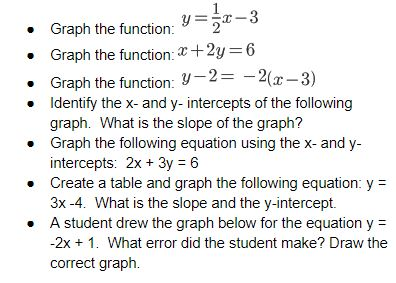2.5 The student demonstrates no major errors or omissions regarding the simpler details and processes (Score 2.0 content) and partial knowledge of the more complex ideas and processes (Score 3.0 content). 2.0 (progressing) There are no major errors or omissions regarding the simpler details and processes as the student can: recognize and recall basic terminology, such as: linear equation. quadratic equation. exponential equation. slope intercepts only interprets and sketches some of the key features of the graph. can identify if a function is linear, exponential, or quadratic However, the student exhibits major errors or omissions regarding the more complex ideas and processes.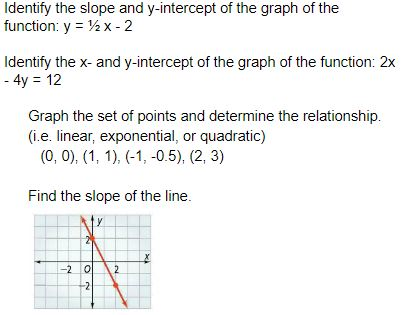1.5 The student demonstrates partial knowledge of the simpler details and processes (Score 2.0 content) but exhibits major errors or omissions regarding the more complex ideas and procedures (Score 3.0 content). 1.0 (beginning) With help, the student demonstrates a partial understanding of some of the simpler details and processes (Score 2.0 content) and some of the more complex ideas and processes (Score 3.0 content). - 0.5 With help, the student demonstrates a partial understanding of some of the simpler details and processes (Score 2.0 content) but not the more complex ideas and processes (Score 3.0 content). 0.0 Even with help, the student demonstrates no understanding or skill. -

## Alg II Graph Quadratic Functions Proficiency Scale

 Score Description Sample Activity 4.0 (advanced) In addition to Score 3.0, the student demonstrates in-depth inferences and applications regarding more complex material that go beyond end of instruction expectations. The graph of  f(x)=ax2+c is shown Points A and B are the same distance from the vertex of the graph of f.  Which point is closer to the vertex of the graph of  f as c increases? Explain your reasoning.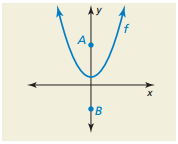3.5 In addition to Score 3.0 performance, the student demonstrates in-depth inferences and applications regarding the more complex content with partial success. 3.0 (proficient) The student can: graph quadratic functions in any form expressed symbolically and show key features of the graph (graph simple cases by hand and use technology to show more complicated cases). The student exhibits no major errors or omissions. Graph the following equations and identify all the key features including:  vertex, x-intercepts, y-intercepts, increasing interval, decreasing interval, maximums, minimums, axis of symmetry,  end behaviors, domain and range.2.5 The student demonstrates no major errors or omissions regarding the simpler details and processes (Score 2.0 content) and partial knowledge of the more complex ideas and processes (Score 3.0 content). 2.0 (progressing) There are no major errors or omissions regarding the simpler details and processes as the student can: recognize and recall basic terminology, such as: quadratic function vertex x- intercepts y- intercepts zeros solutions roots intervals minimum/maximum values axis of symmetry domain range However, the student exhibits major errors or omissions regarding the more complex ideas and processes. Given a graph,  identify all the key features including: vertex,x-intercepts, y-intercepts, increasing interval, decreasing interval, maximums, minimums, axis of symmetry, end behaviors, domain and range. 1.5 The student demonstrates partial knowledge of the simpler details and processes (Score 2.0 content) but exhibits major errors or omissions regarding the more complex ideas and procedures (Score 3.0 content). 1.0 (beginning) With help, the student demonstrates a partial understanding of some of the simpler details and processes (Score 2.0 content) and some of the more complex ideas and processes (Score 3.0 content). 0.5 With help, the student demonstrates a partial understanding of some of the simpler details and processes (Score 2.0 content) but not the more complex ideas and processes (Score 3.0 content). 0.0 Even with help, the student demonstrates no understanding or skill.

## Resources

 Web Vocab intercepts maximum minimum square root function cube root function piecewise-defined function step function absolute value function polynomial function zeroes exponential function

#### MAT-HS.F-IF.07.b

 MAT-HS Targeted Standards(F) Concept: Function(IF) Domain: Interpreting FunctionsCluster: Analyze functions using different representations MAT-HS.F-IF.07* Graph functions expressed symbolically and show key features of the graph, by hand in simple cases and using technology for more complicated cases. a. Graph linear and quadratic functions and show intercepts, maxima, and minima. b. Graph square root, cube root, and piecewise-defined functions, including step functions and absolute value functions. c. Graph polynomial functions, identifying zeros when suitable factorizations are available, and showing end behavior. d. (+) Graph rational functions, identifying zeros and asymptotes when suitable factorizations are available, and showing end behavior. e. Graph exponential and logarithmic functions, showing intercepts and end behavior, and trigonometric functions, showing period, midline, and amplitude. f.  Graph f(x)=sin x and f(x)=cos x as representations of periodic phenomena. g.  (+) Graph trigonometric functions, showing period, midline, phase shift and amplitude

• I can
• I can

• I can
• I can

• I can
• I can

• I can
• I can

## Alg II Graph Square Root Functions Proficiency Scale

Score   Description Sample Activity

4.0

In addition to Score 3.0, the student demonstrates in-depth inferences and applications regarding more complex material that go beyond end of instruction expectations. Graph the following function: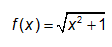3.5 In addition to Score 3.0 performance, the student demonstrates in-depth inferences and applications regarding the more complex content with partial success.

3.0

(proficient)
The student can:
•  graph square root functions symbolically and show key features of the graph (graph simple cases by hand and use technology to show more complicated cases).
The student exhibits no major errors or omissions.
Graph the following equations and identify all the key features including:x-intercepts, y-intercepts, increasing interval, decreasing interval, maximums, minimums, domain and range.2.5 The student demonstrates no major errors or omissions regarding the simpler details and processes (Score 2.0 content) and partial knowledge of the more complex ideas and processes (Score 3.0 content).

2.0

(progressing)

There are no major errors or omissions regarding the simpler details and processes as the student can:
• recognize and recall basic terminology, such as:

• index

• perfect squares

• x-intercepts

• y-intercepts

• increasing intervals

• decreasing intervals

• maximums

• minimums

• domain

• range

However, the student exhibits major errors or omissions regarding the more complex ideas and processes.

 Given the graph, identify all the key features including: x-intercepts, y-intercepts, increasing interval, decreasing interval, maximums, minimums, domain and range.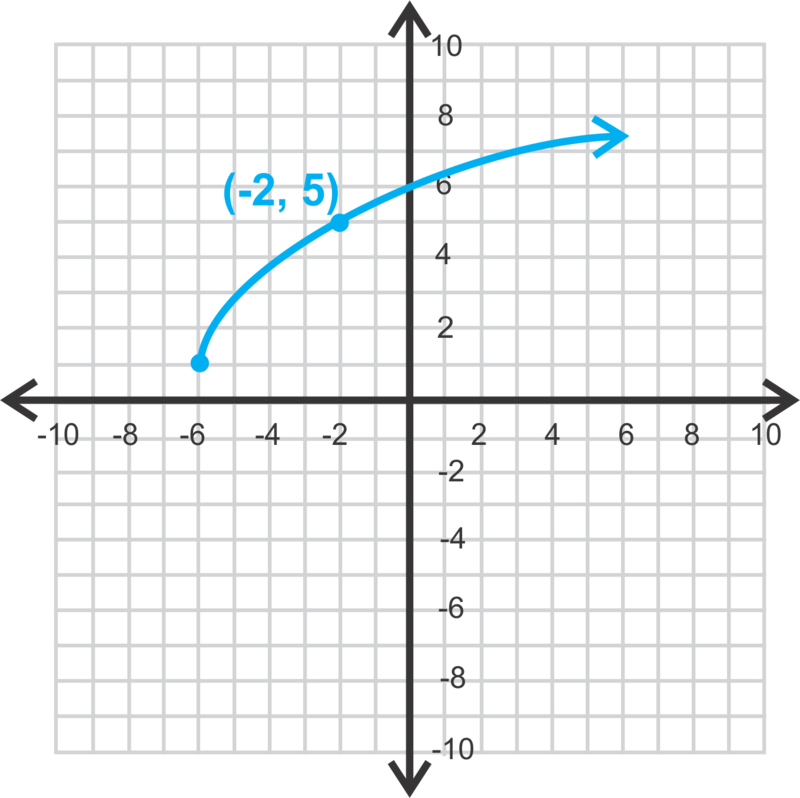1.5 The student demonstrates partial knowledge of the simpler details and processes (Score 2.0 content) but exhibits major errors or omissions regarding the more complex ideas and procedures (Score 3.0 content).

1.0

(beginning)
With help, the student demonstrates a partial understanding of some of the simpler details and processes (Score 2.0 content) and some of the more complex ideas and processes (Score 3.0 content).
0.5 With help, the student demonstrates a partial understanding of some of the simpler details and processes (Score 2.0 content) but not the more complex ideas and processes (Score 3.0 content).
0.0 Even with help, the student demonstrates no understanding or skill.

## Alg II Graph Cube Root Functions Proficiency Scale

Score   Description Sample Activity

4.0

In addition to Score 3.0, the student demonstrates in-depth inferences and applications regarding more complex material that go beyond end of instruction expectations. Explain why your graph of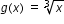is in both the first and third quadrants?
3.5 In addition to Score 3.0 performance, the student demonstrates in-depth inferences and applications regarding the more complex content with partial success.

3.0

(proficient)

The student can:
• graph cube root functions expressed symbolically and show key features of the graph (graph simple cases by hand and use technology to show more complicated cases).
The student exhibits no major errors or omissions.

 Graph the following equations and identify all the key features including: x-intercepts, y-intercepts, increasing interval, decreasing interval, inflection point, domain and range.2.5 The student demonstrates no major errors or omissions regarding the simpler details and processes (Score 2.0 content) and partial knowledge of the more complex ideas and processes (Score 3.0 content).

2.0

(progressing)

There are no major errors or omissions regarding the simpler details and processes as the student can:
• recognize and recall basic terminology, such as:
• index

• perfect cubes

• x-intercepts

• y-intercepts

• increasing intervals

• decreasing intervals

• inflection point

• domain

• range

However, the student exhibits major errors or omissions regarding the more complex ideas and processes.

 Given the graph, identify all the key features including: x-intercepts, y-intercepts, increasing interval, decreasing interval, inflection point, domain and range.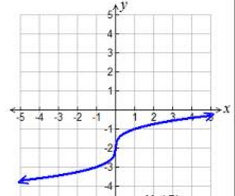1.5 The student demonstrates partial knowledge of the simpler details and processes (Score 2.0 content) but exhibits major errors or omissions regarding the more complex ideas and procedures (Score 3.0 content).

1.0

(beginning)

With help, the student demonstrates a partial understanding of some of the simpler details and processes (Score 2.0 content) and some of the more complex ideas and processes (Score 3.0 content).
0.5 With help, the student demonstrates a partial understanding of some of the simpler details and processes (Score 2.0 content) but not the more complex ideas and processes (Score 3.0 content).
0.0 Even with help, the student demonstrates no understanding or skill.

## Alg II Graph Piecewise Functions Proficiency Scale

Score   Description Sample Activity

4.0

In addition to Score 3.0, the student demonstrates in-depth inferences and applications regarding more complex material that go beyond end of instruction expectations.

 Write the equation of the following piecewise graph and determine the domain and range of the following graph.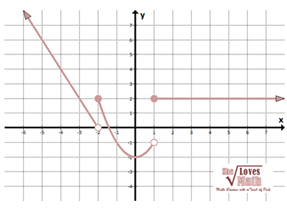3.5 In addition to Score 3.0 performance, the student demonstrates in-depth inferences and applications regarding the more complex content with partial success.

3.0

(proficient)
The student can:
• graph step functions expressed symbolically and show key features of the graph (graph simple cases by hand and use technology to show more complicated cases).
• graph piecewise functions expressed symbolically and show key features of the graph (graph simple cases by hand and use technology to show more complicated cases).

The student exhibits no major errors or omissions.
Graph the following equations and identify all the key features including: increasing interval, decreasing interval, discontinuity, continuity, domain and range.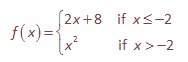2.5 The student demonstrates no major errors or omissions regarding the simpler details and processes (Score 2.0 content) and partial knowledge of the more complex ideas and processes (Score 3.0 content).

2.0

(progressing)
There are no major errors or omissions regarding the simpler details and processes as the student can:
• recognize and recall basic terminology, such as:
• decreasing intervals

• increasing intervals

• discontinuity

• continuity

• domain

• range

However, the student exhibits major errors or omissions regarding the more complex ideas and processes.

 Given the graph, identify all the key features including: increasing interval, decreasing interval, discontinuity, continuity, domain and range.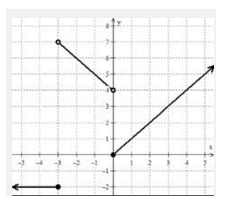1.5 The student demonstrates partial knowledge of the simpler details and processes (Score 2.0 content) but exhibits major errors or omissions regarding the more complex ideas and procedures (Score 3.0 content).

1.0

(beginning)
With help, the student demonstrates a partial understanding of some of the simpler details and processes (Score 2.0 content) and some of the more complex ideas and processes (Score 3.0 content). -
0.5 With help, the student demonstrates a partial understanding of some of the simpler details and processes (Score 2.0 content) but not the more complex ideas and processes (Score 3.0 content).
0.0 Even with help, the student demonstrates no understanding or skill. -

## Alg II Graph Absolute Value Functions Proficiency Scale

Score   Description Sample Activity

4.0

In addition to Score 3.0, the student demonstrates in-depth inferences and applications regarding more complex material that go beyond end of instruction expectations. Graph the following function and explain why the graph looks as it does: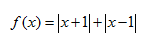3.5 In addition to Score 3.0 performance, the student demonstrates in-depth inferences and applications regarding the more complex content with partial success.

3.0

(proficient)
The student can:
• graph absolute value functions expressed symbolically and show key features of the graph (graph simple cases by hand and use technology to show more complicated cases).

The student exhibits no major errors or omissions.
Graph the following equations and identify all the key features including: vertex, x-intercepts, y-intercepts, increasing interval, decreasing interval, maximums, minimums, domain and range.2.5 The student demonstrates no major errors or omissions regarding the simpler details and processes (Score 2.0 content) and partial knowledge of the more complex ideas and processes (Score 3.0 content).

2.0

(progressing)
There are no major errors or omissions regarding the simpler details and processes as the student can:
• recognize and recall basic terminology, such as:
• absolute value

• vertex

• x-intercepts

• y-intercepts

• intervals (increasing and decreasing)

• maximums

• minimums

• domain

• range

However, the student exhibits major errors or omissions regarding the more complex ideas and processes.

 Given the graph, identify all the key features including: vertex,x-intercepts, y-intercepts, increasing interval, decreasing interval, maximums, minimums, domain and range.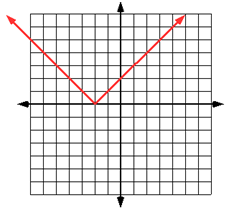1.5 The student demonstrates partial knowledge of the simpler details and processes (Score 2.0 content) but exhibits major errors or omissions regarding the more complex ideas and procedures (Score 3.0 content).

1.0

(beginning)
With help, the student demonstrates a partial understanding of some of the simpler details and processes (Score 2.0 content) and some of the more complex ideas and processes (Score 3.0 content).
0.5 With help, the student demonstrates a partial understanding of some of the simpler details and processes (Score 2.0 content) but not the more complex ideas and processes (Score 3.0 content).
0.0 Even with help, the student demonstrates no understanding or skill.

## Resources

 Web Vocab

#### MAT-HS.F-IF.07.c

 MAT-HS Targeted Standards(F) Concept: Function(IF) Domain: Interpreting FunctionsCluster: Analyze functions using different representations MAT-HS.F-IF.07* Graph functions expressed symbolically and show key features of the graph, by hand in simple cases and using technology for more complicated cases. a. Graph linear and quadratic functions and show intercepts, maxima, and minima. b. Graph square root, cube root, and piecewise-defined functions, including step functions and absolute value functions. c. Graph polynomial functions, identifying zeros when suitable factorizations are available, and showing end behavior. d. (+) Graph rational functions, identifying zeros and asymptotes when suitable factorizations are available, and showing end behavior. e. Graph exponential and logarithmic functions, showing intercepts and end behavior, and trigonometric functions, showing period, midline, and amplitude. f.  Graph f(x)=sin x and f(x)=cos x as representations of periodic phenomena. g.  (+) Graph trigonometric functions, showing period, midline, phase shift and amplitude

• I can
• I can

• I can
• I can

• I can
• I can

• I can
• I can

## Alg II Graph Polynomial Functions Proficiency Scale

 Score Description Sample Activity 4.0 (advanced) In addition to Score 3.0, the student demonstrates in-depth inferences and applications regarding more complex material that go beyond end of instruction expectations. Compare and contrast the two polynomial functions key features.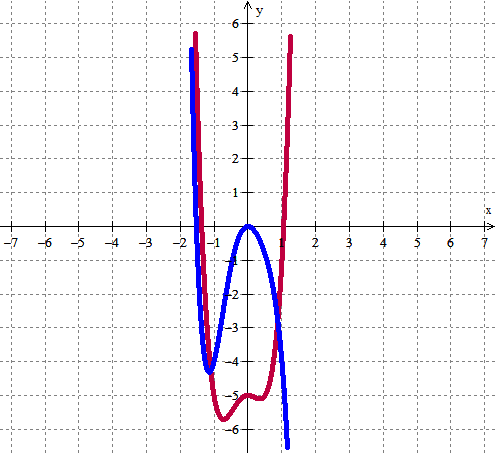3.5 In addition to Score 3.0 performance, the student demonstrates in-depth inferences and applications regarding the more complex content with partial success. 3.0 (proficient) The student can: graph polynomial functions expressed symbolically and show key features of the graph (graph simple cases by hand and use technology to show more complicated cases). identify zeros of a polynomial function when factorable and show end behavior.  The student exhibits no major errors or omissions. Graph the following equations and identify all the key features including:x-intercepts, y-intercepts, increasing interval, decreasing interval, maximums, minimums, end behaviors, domain and range.2.5 The student demonstrates no major errors or omissions regarding the simpler details and processes (Score 2.0 content) and partial knowledge of the more complex ideas and processes (Score 3.0 content). 2.0 (progressing) There are no major errors or omissions regarding the simpler details and processes as the student can: recognize and recall basic terminology, such as: Intervals Increasing Decreasing Minimum Maximum End behavior Terms Factors Lead Coefficient Constant Degree Cubic Quartic Polynomial Domain Range            However, the student exhibits major errors or omissions regarding the more complex ideas and processes. Given the graph, identify all the key features including: x-intercepts, y-intercepts, increasing interval, decreasing interval, maximums, minimums, end behaviors, domain and range.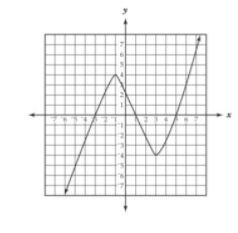1.5 The student demonstrates partial knowledge of the simpler details and processes (Score 2.0 content) but exhibits major errors or omissions regarding the more complex ideas and procedures (Score 3.0 content). 1.0 (beginning) With help, the student demonstrates a partial understanding of some of the simpler details and processes (Score 2.0 content) and some of the more complex ideas and processes (Score 3.0 content). - 0.5 With help, the student demonstrates a partial understanding of some of the simpler details and processes (Score 2.0 content) but not the more complex ideas and processes (Score 3.0 content). 0.0 Even with help, the student demonstrates no understanding or skill. -

## Resources

 Web Vocab

#### MAT-HS.F-IF.07.d

 MAT-HS Targeted Standards(F) Concept: Function(IF) Domain: Interpreting FunctionsCluster: Analyze functions using different representations MAT-HS.F-IF.07 Graph functions expressed symbolically and show key features of the graph, by hand in simple cases and using technology for more complicated cases. a. Graph linear and quadratic functions and show intercepts, maxima, and minima. b. Graph square root, cube root, and piecewise-defined functions, including step functions and absolute value functions. c. Graph polynomial functions, identifying zeros when suitable factorizations are available, and showing end behavior. d. (+) Graph rational functions, identifying zeros and asymptotes when suitable factorizations are available, and showing end behavior. e. Graph exponential and logarithmic functions, showing intercepts and end behavior, and trigonometric functions, showing period, midline, and amplitude. f.  Graph f(x)=sin x and f(x)=cos x as representations of periodic phenomena. g.  (+) Graph trigonometric functions, showing period, midline, phase shift and amplitude

• I can
• I can

• I can
• I can

• I can
• I can

• I can
• I can

## Proficiency Scale

 Score Description Sample Activity 4.0 (advanced proficient) In addition to Score 3.0, the student demonstrates in-depth inferences and applications regarding more complex material that go beyond end of instruction expectations. -Student is able to determine and describe intervals where the function is increasing and decreasing using interval notation. 3.5 In addition to Score 3.0 performance, the student demonstrates in-depth inferences and applications regarding the more complex content with partial success. 3.0 (proficient) The student can:   The student exhibits no major errors or omissions. 2.5 The student demonstrates no major errors or omissions regarding the simpler details and processes (Score 2.0 content) and partial knowledge of the more complex ideas and processes (Score 3.0 content). 2.0 (developing) There are no major errors or omissions regarding the simpler details and processes as the student can: recognize and recall basic terminology, such as: However, the student exhibits major errors or omissions regarding the more complex ideas and processes. 1.5 The student demonstrates partial knowledge of the simpler details and processes (Score 2.0 content) but exhibits major errors or omissions regarding the more complex ideas and procedures (Score 3.0 content). 1.0 (novice) With help, the student demonstrates a partial understanding of some of the simpler details and processes (Score 2.0 content) and some of the more complex ideas and processes (Score 3.0 content). - 0.5 With help, the student demonstrates a partial understanding of some of the simpler details and processes (Score 2.0 content) but not the more complex ideas and processes (Score 3.0 content). 0.0 Even with help, the student demonstrates no understanding or skill. -

## Resources

 Web Vocab

#### MAT-HS.F-IF.07.e

 MAT-HS Targeted Standards(F) Concept: Function(IF) Domain: Interpreting FunctionsCluster: Analyze functions using different representations MAT-HS.F-IF.07* Graph functions expressed symbolically and show key features of the graph, by hand in simple cases and using technology for more complicated cases. a. Graph linear and quadratic functions and show intercepts, maxima, and minima. b. Graph square root, cube root, and piecewise-defined functions, including step functions and absolute value functions. c. Graph polynomial functions, identifying zeros when suitable factorizations are available, and showing end behavior. d. (+) Graph rational functions, identifying zeros and asymptotes when suitable factorizations are available, and showing end behavior. e. Graph exponential and logarithmic functions, showing intercepts and end behavior, and trigonometric functions, showing period, midline, and amplitude. f.  Graph f(x)=sin x and f(x)=cos x as representations of periodic phenomena. g.  (+) Graph trigonometric functions, showing period, midline, phase shift and amplitude

## Student Learning Targets:

• I can
• I can

• I can
• I can

### Skills (Performance) Targets

• Given an equation, I can graph a function and label all critical points, e.g. vertex, max or min value, x-intercepts, y-intercepts, slope.
• I can graph functions expressed symbolically and show key features of the graph (graph simple cases by hand and use technology to show more complicated cases).
• I can graph exponential functions, showing intercepts and end behavior.

• I can
• I can

## Proficiency Scale

 Score Description Sample Activity 4.0 In addition to Score 3.0, the student demonstrates in-depth inferences and applications regarding more complex material that go beyond end of instruction expectations. -Student is able to determine and describe intervals where the function is increasing and decreasing using interval notation. 3.5 In addition to Score 3.0 performance, the student demonstrates in-depth inferences and applications regarding the more complex content with partial success. 3.0 The student can: interprets and sketches key features of the linear graph (HS.F-IF.7a1) including: intercepts positive/negative slope interprets and sketches key features of the exponential graph (HS.F-IF.7b2) including: end behavior (growth/decay) interprets and sketches key features of the quadratic graph (HS.F-IF.7e) including: minimum/maximum axis of symmetry intercepts construct simple graphs by hand and use technology for more complicated cases. The student exhibits no major errors or omissions. Graph the function and identify the vertex, intercepts, and axis of symmetry.  y=x2-8x-7 Graph the function and identify the end behavior of the graph. y=(1/2)x 2.5 The student demonstrates no major errors or omissions regarding the simpler details and processes (Score 2.0 content) and partial knowledge of the more complex ideas and processes (Score 3.0 content). 2.0 There are no major errors or omissions regarding the simpler details and processes as the student can: recognize and recall basic terminology, such as: linear equation. quadratic equation. exponential equation. slope intercepts minimum maximum end behavior growth and decay symmetry axis of symmetry only interprets and sketches some of the key features of the graph. can identify if a function is linear, exponential, or quadratic However, the student exhibits major errors or omissions regarding the more complex ideas and processes. Graph the set of points and determine the relationship.  (i.e. linear, exponential, or quadratic)   (0, 0), (1, 1), (-1, -0.5), (2, 3) 1.5 The student demonstrates partial knowledge of the simpler details and processes (Score 2.0 content) but exhibits major errors or omissions regarding the more complex ideas and procedures (Score 3.0 content). 1.0 With help, the student demonstrates a partial understanding of some of the simpler details and processes (Score 2.0 content) and some of the more complex ideas and processes (Score 3.0 content). - 0.5 With help, the student demonstrates a partial understanding of some of the simpler details and processes (Score 2.0 content) but not the more complex ideas and processes (Score 3.0 content). 0.0 Even with help, the student demonstrates no understanding or skill. -

## Alg II Graph Exponential Functions Proficiency Scale

 Score Description Sample Activity 4.0 (advanced) In addition to Score 3.0, the student demonstrates in-depth inferences and applications regarding more complex material that go beyond end of instruction expectations. Write an exponential function whose graph lies between the graphs of y=(0.5)x and y=(0.25)x+3 3.5 In addition to Score 3.0 performance, the student demonstrates in-depth inferences and applications regarding the more complex content with partial success. 3.0 (proficient) The student can: graph functions expressed symbolically and show key features of the graph (graph simple cases by hand and use technology to show more complicated cases). graph exponential functions, showing intercepts and end behavior (asymptote). The student exhibits no major errors or omissions. Graph the following equations and identify all the key features including: x-intercepts, y-intercepts, increasing interval, decreasing interval, domain, range and asymptote.2.5 The student demonstrates no major errors or omissions regarding the simpler details and processes (Score 2.0 content) and partial knowledge of the more complex ideas and processes (Score 3.0 content). 2.0 (progressing) There are no major errors or omissions regarding the simpler details and processes as the student can: recognize and recall basic terminology, such as: x-intercepts y-intercepts intervals (decreasing and increasing) asymptote domain range However, the student exhibits major errors or omissions regarding the more complex ideas and processes. Given the graph, identify all the key features including: x-intercepts, y-intercepts, increasing interval, decreasing interval, domain and range and asymptote.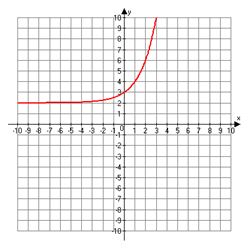1.5 The student demonstrates partial knowledge of the simpler details and processes (Score 2.0 content) but exhibits major errors or omissions regarding the more complex ideas and procedures (Score 3.0 content). 1.0 (beginning) With help, the student demonstrates a partial understanding of some of the simpler details and processes (Score 2.0 content) and some of the more complex ideas and processes (Score 3.0 content). 0.5 With help, the student demonstrates a partial understanding of some of the simpler details and processes (Score 2.0 content) but not the more complex ideas and processes (Score 3.0 content). 0.0 Even with help, the student demonstrates no understanding or skill.

## Alg II Graph Logarithmic Functions Proficiency Scale

Score   Description Sample Activity

4.0

In addition to Score 3.0, the student demonstrates in-depth inferences and applications regarding more complex material that go beyond end of instruction expectations. Once a hurricane reaches land, the wind speed s (in knots) within the hurricane is related to the time t (in hours) the hurricane remains over land.  For one particular hurricane, this relationship can be modeled  by the equation y= -57.1 log t +121. Graph the equation and analyze the graph to determine how long the hurricane must be over land for winds speeds to become about 80 knots.
3.5 In addition to Score 3.0 performance, the student demonstrates in-depth inferences and applications regarding the more complex content with partial success.

3.0

(proficient)

The student can:
• Graph logarithmic functions expressed symbolically and show key features of the graph (graph simple cases by hand and use technology to show more complicated cases).

The student exhibits no major errors or omissions.

Graph the following equation and identify all the key features including: increasing interval, decreasing interval, asymptote, domain and range.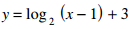2.5 The student demonstrates no major errors or omissions regarding the simpler details and processes (Score 2.0 content) and partial knowledge of the more complex ideas and processes (Score 3.0 content).

2.0

(progressing)

There are no major errors or omissions regarding the simpler details and processes as the student can:
• recognize and recall basic terminology, such as:
• logarithm
• base
• exponent
• intervals (increasing and decreasing)
• asymptote
• domain
• range

However, the student exhibits major errors or omissions regarding the more complex ideas and processes.

 Given the graph, identify all the key features including the asymptote, increasing and decreasing intervals, domain, and range.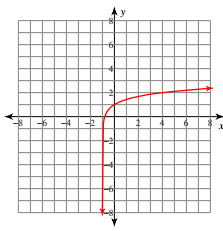1.5 The student demonstrates partial knowledge of the simpler details and processes (Score 2.0 content) but exhibits major errors or omissions regarding the more complex ideas and procedures (Score 3.0 content).

1.0

(beginning)

With help, the student demonstrates a partial understanding of some of the simpler details and processes (Score 2.0 content) and some of the more complex ideas and processes (Score 3.0 content).
0.5 With help, the student demonstrates a partial understanding of some of the simpler details and processes (Score 2.0 content) but not the more complex ideas and processes (Score 3.0 content).
0.0 Even with help, the student demonstrates no understanding or skill.

## Resources

 Web Vocab intercepts maximum asymptote minimum exponential function

#### MAT-HS.F-IF.07.f

 MAT-HS Targeted Standards(F) Concept: Function(IF) Domain: Interpreting FunctionsCluster: Analyze functions using different representations MAT-HS.F-IF.07* Graph functions expressed symbolically and show key features of the graph, by hand in simple cases and using technology for more complicated cases. a. Graph linear and quadratic functions and show intercepts, maxima, and minima. b. Graph square root, cube root, and piecewise-defined functions, including step functions and absolute value functions. c. Graph polynomial functions, identifying zeros when suitable factorizations are available, and showing end behavior. d. (+) Graph rational functions, identifying zeros and asymptotes when suitable factorizations are available, and showing end behavior. e. Graph exponential and logarithmic functions, showing intercepts and end behavior, and trigonometric functions, showing period, midline, and amplitude. f.  Graph f(x)=sin x and f(x)=cos x as representations of periodic phenomena. g.  (+) Graph trigonometric functions, showing period, midline, phase shift and amplitude

• I can
• I can

• I can
• I can

• I can
• I can
• I can

• I can
• I can

## Alg II Graph Sine and Cosine Functions Proficiency Scale

Score   Description Sample Activity

4.0

In addition to Score 3.0, the student demonstrates in-depth inferences and applications regarding more complex material that go beyond end of instruction expectations. Write an equation for the graph in the form y = A sin ( Bx + C ). Write an equation for the same graph in the form y = A cos( Bx+C).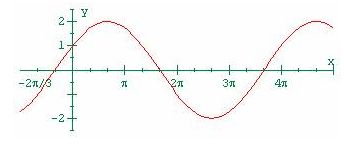3.5 In addition to Score 3.0 performance, the student demonstrates in-depth inferences and applications regarding the more complex content with partial success.

3.0

(proficient)

The student can:
• graph trigonometric functions expressed symbolically and show key features of the graph (graph simple cases by hand and use technology to show more complicated cases)

The student exhibits no major errors or omissions.
Graph the following equations and identify all the key features including: increasing interval, decreasing interval, maximums, minimums, domain and range, amplitude, increment, period, midline. f(x) = asinx

2.5 The student demonstrates no major errors or omissions regarding the simpler details and processes (Score 2.0 content) and partial knowledge of the more complex ideas and processes (Score 3.0 content).

2.0

(progressing)

There are no major errors or omissions regarding the simpler details and processes as the student can:

• recognize and recall basic terminology, such as:

• trigonometric function

• amplitude

• increment

• period

• midline

• domain

• range

However, the student exhibits major errors or omissions regarding the more complex ideas and processes.

 Given the graph, identify all the key features including: increasing interval, decreasing interval, maximums, minimums, domain and range, amplitude, increment, period,and midline.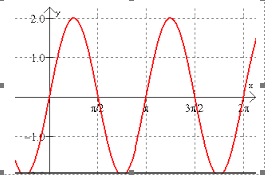1.5 The student demonstrates partial knowledge of the simpler details and processes (Score 2.0 content) but exhibits major errors or omissions regarding the more complex ideas and procedures (Score 3.0 content).

1.0

(beginning)

With help, the student demonstrates a partial understanding of some of the simpler details and processes (Score 2.0 content) and some of the more complex ideas and processes (Score 3.0 content).
0.5 With help, the student demonstrates a partial understanding of some of the simpler details and processes (Score 2.0 content) but not the more complex ideas and processes (Score 3.0 content).
0.0 Even with help, the student demonstrates no understanding or skill.

## Resources

 Web Vocab

#### MAT-HS.F-IF.07.g

 MAT-HS Targeted Standards(F) Concept: Function(IF) Domain: Interpreting FunctionsCluster: Analyze functions using different representations MAT-HS.F-IF.07 Graph functions expressed symbolically and show key features of the graph, by hand in simple cases and using technology for more complicated cases. a. Graph linear and quadratic functions and show intercepts, maxima, and minima. b. Graph square root, cube root, and piecewise-defined functions, including step functions and absolute value functions. c. Graph polynomial functions, identifying zeros when suitable factorizations are available, and showing end behavior. d. (+) Graph rational functions, identifying zeros and asymptotes when suitable factorizations are available, and showing end behavior. e. Graph exponential and logarithmic functions, showing intercepts and end behavior, and trigonometric functions, showing period, midline, and amplitude. f.  Graph f(x)=sin x and f(x)=cos x as representations of periodic phenomena. g.  (+) Graph trigonometric functions, showing period, midline, phase shift and amplitude

• I can
• I can

• I can
• I can

• I can
• I can

• I can
• I can

## Proficiency Scale

 Score Description Sample Activity 4.0 In addition to Score 3.0, the student demonstrates in-depth inferences and applications regarding more complex material that go beyond end of instruction expectations. -Student is able to determine and describe intervals where the function is increasing and decreasing using interval notation. 3.5 In addition to Score 3.0 performance, the student demonstrates in-depth inferences and applications regarding the more complex content with partial success. 3.0 The student can:   The student exhibits no major errors or omissions. 2.5 The student demonstrates no major errors or omissions regarding the simpler details and processes (Score 2.0 content) and partial knowledge of the more complex ideas and processes (Score 3.0 content). 2.0 There are no major errors or omissions regarding the simpler details and processes as the student can: recognize and recall basic terminology, such as: However, the student exhibits major errors or omissions regarding the more complex ideas and processes. 1.5 The student demonstrates partial knowledge of the simpler details and processes (Score 2.0 content) but exhibits major errors or omissions regarding the more complex ideas and procedures (Score 3.0 content). 1.0 With help, the student demonstrates a partial understanding of some of the simpler details and processes (Score 2.0 content) and some of the more complex ideas and processes (Score 3.0 content). - 0.5 With help, the student demonstrates a partial understanding of some of the simpler details and processes (Score 2.0 content) but not the more complex ideas and processes (Score 3.0 content). 0.0 Even with help, the student demonstrates no understanding or skill. -

## Resources

 Web Vocab

#### MAT-HS.F-IF.08

 MAT-HS Targeted Standards(F) Concept: Function(IF) Domain: Interpreting FunctionsCluster: Analyze functions using different representations MAT-HS.F-IF.08 Write a function defined by an expression in different but equivalent forms to reveal and explain different properties of the function. a. Use the process of factoring and completing the square in a quadratic function to show zeros, extreme values, and symmetry of the graph, and interpret these in terms of a context. b. Use the properties of exponents to interpret expressions for exponential functions. For example, identify percent rate of change in functions such as y = (1.02)t, y = (0.97)t, y = (1.01)12t, y = (1.2)t/10, and classify them as representing exponential growth or decay.

## Student Learning Targets:

### Knowledge Targets

• I can recall the order of operations.
• I can understand and identify a power and its parts (base and exponent).
• I can recognize the properties of exponents (i.e. product of powers, power of a power, power of a product).
• I can recognize a zero or negative exponent.
• I can identify a radical.
• I can identify if a radical expression is in simplest form.
• I can recognize the properties of radicals, i.e., product, quotient, and sum of radicals.
• I can identify perfect squares.
• I can define a logarithm.
• I can recall properties of logarithms and exponents.
• I can recognize common logarithms and natural logarithms.
• I can identify an asymptote.
• I can recall the change of base formula.

### Reasoning Targets

• I can analyze an algebraic expression.
• I can decide what to do next based on my knowledge of the order of operations.
• I can distinguish the difference of when to use each property of radicals.
• I can evaluate the properties of radicals.
• I can apply the distributive property.
• I can analyze what type of factoring technique that should be used for a given expression. (i.e. factor trinomials, factor difference of squares, factor sum and difference of cubes, factor by grouping, factor out the greatest common factor)
• I can combine like terms in an expression or equation.
• I can convert from a logarithm to exponential expressions.
• I can identify an asymptote.
• I can expand and condense logarithms.
• I can evaluate logarithms and exponential expressions.
• I can distinguish between a natural log and common log.

### Skills (Performance) Targets

• I can simplify an algebraic expression.
• I can apply factor using perfect square.
• I can do prime factorization.
• I can rationalize a denominator.
• I can simplify a polynomial expression.
• I can multiply polynomials.
• I can justify the steps taken in manipulating an expression.
• I can complete all necessary steps in order to simplify the expression.
• I can distribute and factor an expression.
• I can solve for (isolate) a specific variable.
• I can perform transformations that produce equivalent equations (e.g., adding the same amount to both sides of the equation, etc.).
• I can check the solution.
• I can set a polynomial equal to zero and solve by factoring, quadratic formula, graphing, linear combinations, and substitution.
• I can solve for (isolate) a specific variable.
• I can write a linear system.
• I can perform transformations that produce equivalent equations (e.g., adding the same amount to both sides of the equation, etc.)
• I can check the solution.
• I can solve a quadratic.
• I can multiply and factor polynomials.
• I can solve a linear inequality.
• I can graph a linear inequality.
• I can solve a system of linear inequalities.
• I can graph a system of linear inequalities.
• I can check the solution of the system.
• I can solve a linear system (substitution, linear combination, graphically).
• I can write a linear system.
• I can identify the number of solutions of a linear system.
• I can identify the most efficient method to solve a system.
• I can check the solution of the system.
• I can apply basic properties of exponents and logarithms to rewrite algebraic expressions.
• I can graph logarithmic and exponential functions.
• I can solve logarithmic and exponential equations.
• I can apply the change of base formula to evaluate a logarithmic expression.
• I can graph and determine the equation of an asymptote.
• I can write a function in equivalent forms (eg. factored vs. general form) to show different properties of the function.
• I can explain different properties of a function that are revealed by writing a function in equivalent forms.
• I can use the process of factoring and completing the square in a quadratic function to show zeros, a maximum or minimum, and symmetry of the graph, and interpret these in terms of a real-world situation.
• I can use the properties of exponents to interpret exponential functions as growth or decay.

• I can
• I can

## Proficiency Scale

 Score Description Sample Activity 4.0 In addition to achieving level 3.0 content, the student makes in-depth inferences and applications that go beyond what was taught - 3.5 In addition to Score 3.0 performance, the student demonstrates in-depth inferences and applications regarding the more complex content with partial success. 3.0 The student can: Write a function in equivalent forms (eg. factored vs. general form) to show different properties of the function Explain different properties of a function that are revealed by writing a function in equivalent forms Use the process of factoring and completing the square in a quadratic function to show zeros, a maximum or minimum, and symmetry of the graph, and interpret these in terms of a real-world situation Use the properties of exponents to interpret exponential functions as growth or decay The student exhibits no major errors or omissions. Given f(x)=x2-5x-6: Rewrite the function in factored form to find the zeros.    Find the x value of the maximum or minimum and state if a maximum or minimum occurs at that x value. 2.5 The student demonstrates no major errors or omissions regarding the simpler details and processes (Score 2.0 content) and partial knowledge of the more complex ideas and processes (Score 3.0 content). 2.0 There are no major errors or omissions regarding the simpler details and processes as the student: recognizes and recalls specific terminology, such as exponential growth exponential decay However, the student exhibits major errors or omissions regarding the more complex ideas and processes. - 1.5 The student demonstrates partial knowledge of the simpler details and processes (Score 2.0 content) but exhibits major errors or omissions regarding the more complex ideas and procedures (Score 3.0 content). 1.0 With help, a partial understanding of some of the simpler details and processes and some of the more complex ideas and processes. - 0.5 With help, the student demonstrates a partial understanding of some of the simpler details and processes (Score 2.0 content) but not the more complex ideas and processes (Score 3.0 content).

## Alg II Equivalent Quadratic Expressions Proficiency Scale

 Score Description Sample Activity 4.0 (advanced) In addition to Score 3.0, the student demonstrates in-depth inferences and applications regarding more complex material that go beyond end of instruction expectations. Write the equation of the following graph in the three forms.  If you cannot write the function in one of the forms, explain why.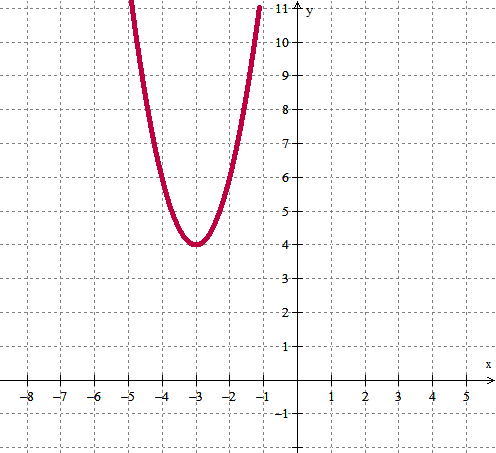3.5 In addition to Score 3.0 performance, the student demonstrates in-depth inferences and applications regarding the more complex content with partial success. 3.0 (proficient) The student can: explain the different properties of a function that are revealed by writing a function in equivalent forms. write a function in equivalent forms (factored vs. general form) to show different properties of the function. The student exhibits no major errors or omissions. Rewrite the following equation of the parabola in vertex form.  Identify the minimum/maximum value. f(x)=2x2+16x+10   Rewrite the following equation of the parabola in standard form and find the vertex.f(x)=2(2x-1)(x+9)  Explain the advantages of each form of a quadratic function. 2.5 The student demonstrates no major errors or omissions regarding the simpler details and processes (Score 2.0 content) and partial knowledge of the more complex ideas and processes (Score 3.0 content). 2.0 (progressing) There are no major errors or omissions regarding the simpler details and processes as the student can: identify the different forms of functions (eg. Factored vs. general form) that show different properties of the function. recognize and recall basic terminology, such as: factor intercept vertex complete the square standard form parabola However, the student exhibits major errors or omissions regarding the more complex ideas and processes. Label each of the following functions with the quadratic form it represents. f(x)=2x2+5x+9 q(x)=3(2x+5)(x-2)    3. g(x)=4(x-3)2+6 1.5 The student demonstrates partial knowledge of the simpler details and processes (Score 2.0 content) but exhibits major errors or omissions regarding the more complex ideas and procedures (Score 3.0 content). 1.0 (beginning) With help, the student demonstrates a partial understanding of some of the simpler details and processes (Score 2.0 content) and some of the more complex ideas and processes (Score 3.0 content). 0.5 With help, the student demonstrates a partial understanding of some of the simpler details and processes (Score 2.0 content) but not the more complex ideas and processes (Score 3.0 content). 0.0 Even with help, the student demonstrates no understanding or skill.

## Resources

 Web Vocab exponential growth exponential decay

Page: (Previous)   1  2  3  4  (Next)
ALL